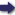(London :  Kegan Paul, Trench, Trübner & Co.,  1910.)

 Tools

## Search this bookPrev Page 142 Next``` 142 ALBERUNPS INDIA. but his calculation showing how to find that place which every single foot occupies in this series of per¬ mutations is not in accordance with it. For he says: "Place the numeral 2 to denote each element of a foot (i.e. both guru and laghu), once for all, so that every foot is represented by 2, 2, 2. Multiply the left (number) by the middle, and the product by the right one. If this multiplier (i.e. this number of the right side) is a laghu, then leave the product as it is ; but if it is a guru, subtract one from the product." The author exemplifies this with the sixth foot, i.e. 1 < |. He multiplies 2 by 2, and from the product (4) he subtracts i. The remaining 3 he multiplies by the third 2, aud he gets the product of 6. This, however, is not correct for most of the feet, and I am rather inclined to believe that the text of the manuscript is corrupt. The proper order of the feet would accordingly be the following: III. I. II. III. I. < < < 2. 1 < < 3- < 1 < 4- 1 1 < I. n. 5- < < 6. 1 < 7- < 1 The mixture of the first line (No. I.) is such that one kind always follows the other. In the second line (No, II.) two of one kind are followed by two of the other; and in the third line (No. III.) four of one kind are followed by four of the other. Then the author of the above-mentioned calculation goes on to say : " If the first element of the foot is a guru, subtract one before you multiply. If the multi¬ plier is a guru, subtract one from the product. Thus you find the place which a foot occupies in this order." Onthe As the Arabic verse is divided into two halves or ^^ "^' hemistichs by the drud, i.e. the last foot of the first ```Prev Page 142 Next